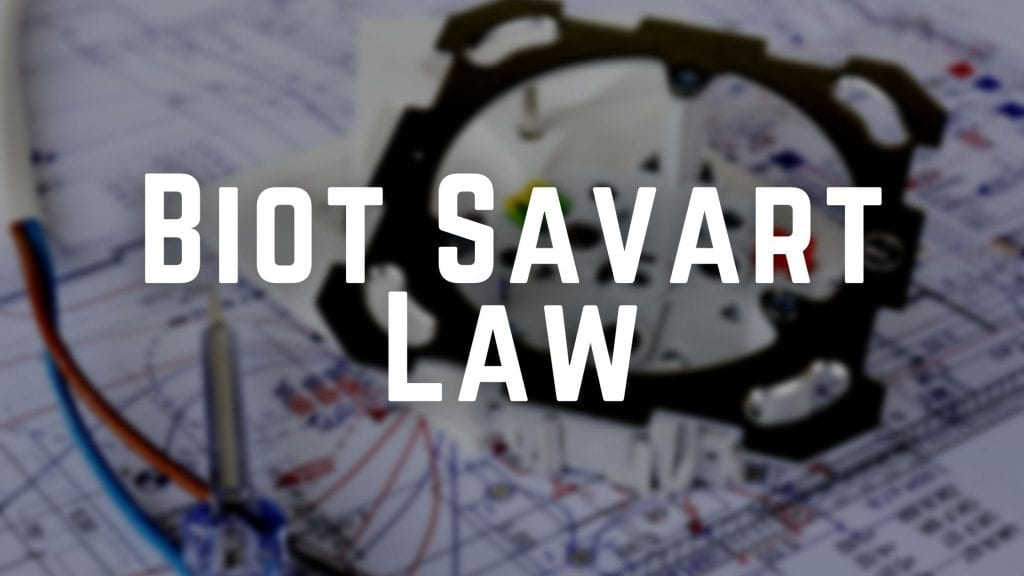Physics

# Biot Savart Law and Magnetic Field Due to Circular Loop

To find out magnetic field at any point on axis of circular loop we use Biot Savart Law. Learn the Biot Savart Law in detail here. Also see derivation of magnetic field on the axis of circular loop (ring).

5 minutes long
Posted by Mahak Jain, 27/5/2021Hesap Oluştur

Got stuck on homework? Get your step-by-step solutions from real tutors in minutes! 24/7. Unlimited.

All magnetic fields that we know are due to current (or moving charges) and due to intrinsic magnetic moments of particles. You might be wondering that what is intrinsic magnetic moments of particles. In short it means magnetic field due to magnets and ferromagnetic materials like iron. We will discuss it in detail next blog of magnets and also in next chapter, magnetism and matter. In this blog we shall study Biot Savart Law. This law gives the relation between current and the magnetic field it produces.

## State Biot Savart Law

According to Biot Savart law, the magnitude of the magnetic field dB is proportional to the current I, the element length |dl|, and inversely proportional to the square of the distance r.

## Biot Savart Law Derivation

Figure below shows a finite conductor XY carrying current I. Consider an differential element dl of the conductor. The magnetic field due to this element is dB, which is to be determined at a point P, which is at a distance r from it. Let θ be the angle between dl and the displacement vector r. Direction of dl is same as direction of current.

According to Biot Savart law, the magnitude of the magnetic field dB is proportional to the current I, the element length |dl|, and inversely proportional to the square of the distance r. Its direction is perpendicular to the plane containing dl and r. This is because formula contains cross product of dl and r vectors. Thus, in vector notation,

On integrating we get the magnitude of this field, which is,

## Similarities and Differences between Coulomb’s Law and Biot Savart Law

The Biot Savart law for the magnetic field has certain similarities with the Coulomb’s law for the electrostatic field. Some of these are:

1. Both are long range, since both depend inversely on the square of distance from the source to the point of interest.
2. The principle of superposition applies to both fields. This is because, both are vectors. So, we can treat them like vectors.

The Biot Savart law for the magnetic field has certain differences with the Coulomb’s law for the electrostatic field. Some of these are:

1. Electric charge, a scalar quantity produce electrostatic field. Whereas, current and length vector (Idl, a vector quantity) produce magnetic field.
2. Electrostatic field is along r vector, whereas magnetic field is perpendicular to r vector.
3. There is an angle dependence in the Biot Savart law which is not present in the case of electrostatic field.

## Formula of Speed of Light

There is an interesting relation between ε0 , the permittivity of free space; µ0 , the permeability of free space; and c, the speed of light in vacuum:

## Magnetic Field Due to a Current Through a Circular Loop

We studied Biot Savart Law, but what is it’s application? Where is it used? Here we find magnetic field at the axis of current carrying circular loop.

Assume a ring of radius R and circumference l. Take a differential element of length dl. Current in ring is I and is to be assumed constant.

The figure above shows a circular loop placed in the y-z plane with its centre at the origin O. The x-axis is the axis of the loop. We wish to calculate the magnetic field at the point P on this axis. Let x be the distance of P from the centre O of the loop.

Applying Biot Savart Law

Every element dl is perpendicular to r vector. So, angle between dl and r is 90°. Also, r2=R2+x2. Thus,

By symmetry perpendicular component of dB will cancel out and only dBx will remain.

Therefore, dBx = dB cosθ

cosθ = R/(R2+x2)1/2

On integrating dl converts to l, and l=2πR

Thus, final expression becomes:

### Special Case

When point P is in the plane of circular loop, that is x=0

B0 = μ0I / 2R